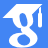Dr. Cristian-Daniel Alecsa

(former researcher at ICTP, between 2017-2021)

This website does not contain the papers elaborated after leaving ICTP.

Last update: February 21, 2022.

•ORCID
••ResearchGate
•Publons Profile
ResearcherID
•Scopus profile
•Former position:

Research Assistant at Tiberiu Popoviciu Institute of Numerical Analysis

Postdoc, Romanian Institute for Science and Technology

Research domains:

1. Optimization Methods for Machine Learning
2. Data Science Techniques
3. Scientific Computing & Numerical Algorithms
4. Mathematical Modeling

## Applications of fibre contraction principle to some classes of functional integral equations

Abstract Let a<c<b real numbers, (B,|·|) a (real or complex) Banach space, H∈C([a,b]×[a,c]×B,B),K∈C([a,b]² ×B,B),g∈C([a,b],B),A:C([a,c],B)→C([a, c], B) and B:C([a,b],B)→C([a,b],B). In this paper we study the following functional integral equation, x(t)=∫_{a}^{c}H(t,s,A(x)(s))ds+∫_{a}^{t}K(t,s,B(x)(s))ds+g(t),t∈[a,b]. By…
Abstract We deal with the existence and localization of positive radial solutions for Dirichlet problems involving $$\phi$$-Laplacian operators in a ball. In particular, $$p$$-Laplacian and Minkowski-curvature equations are considered. Our…